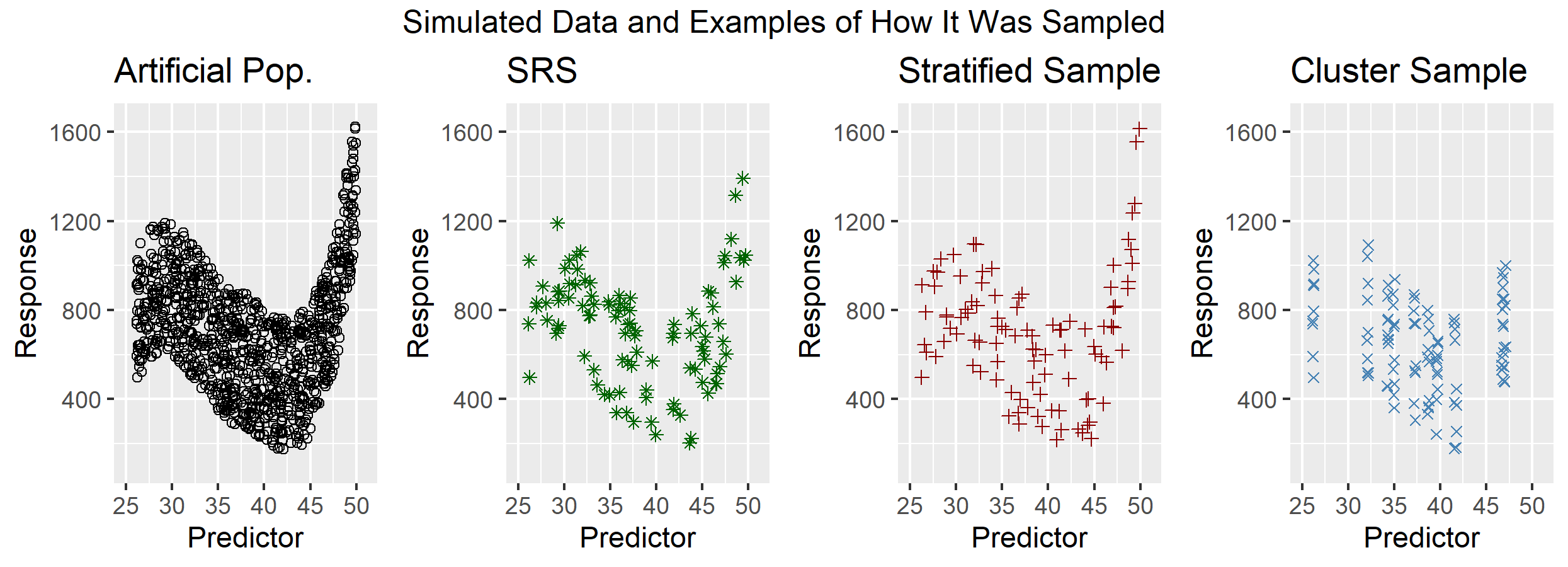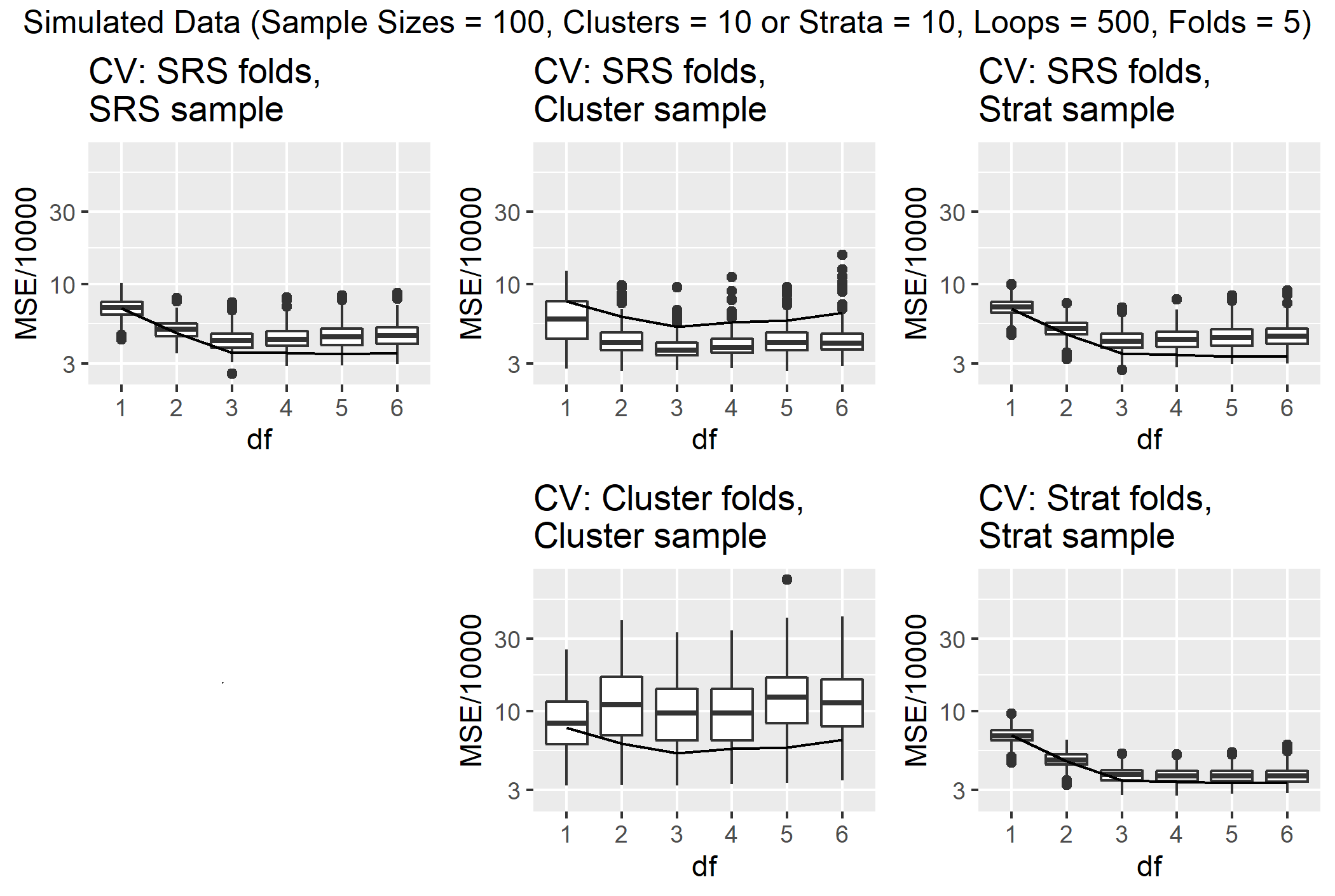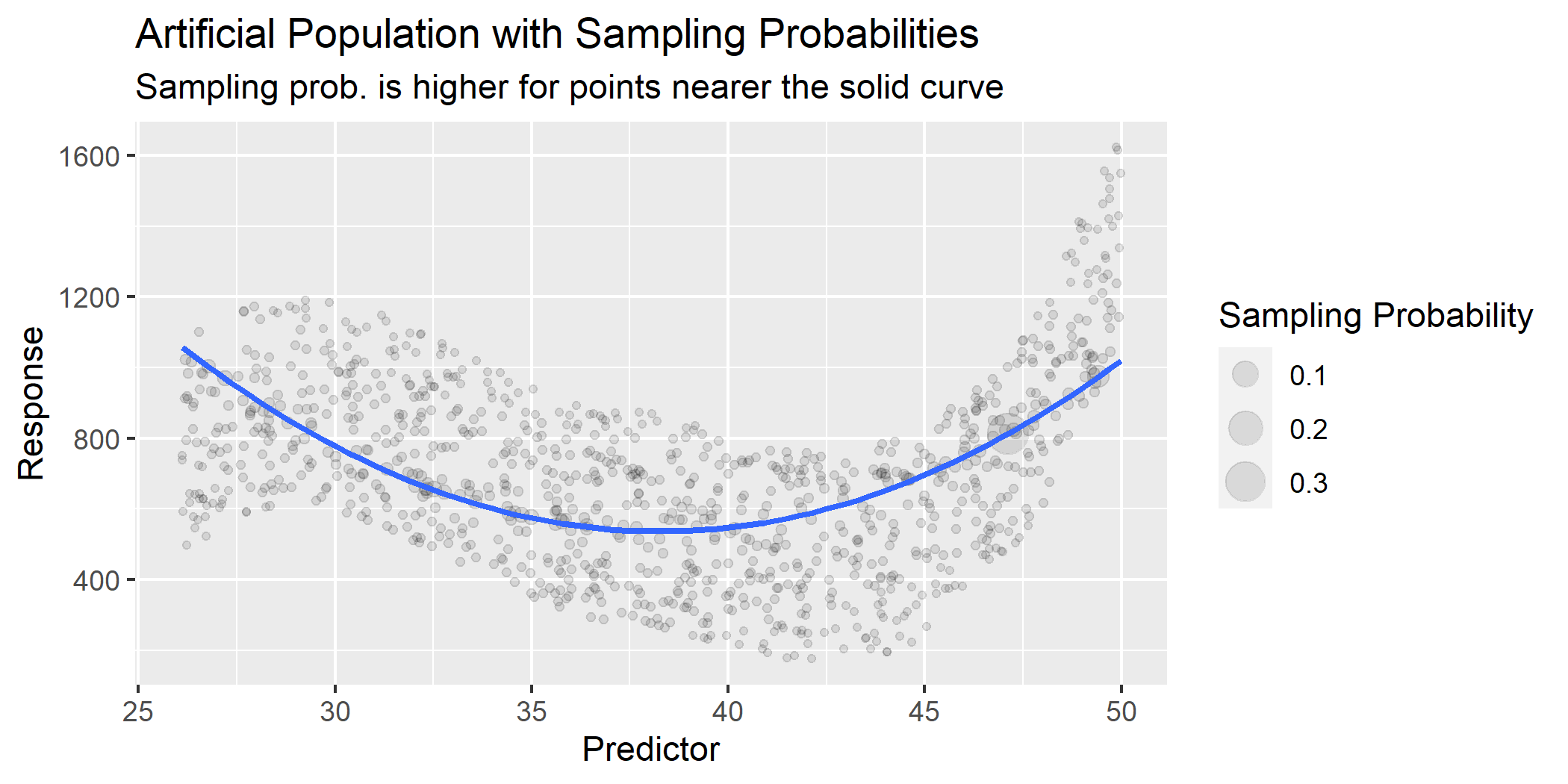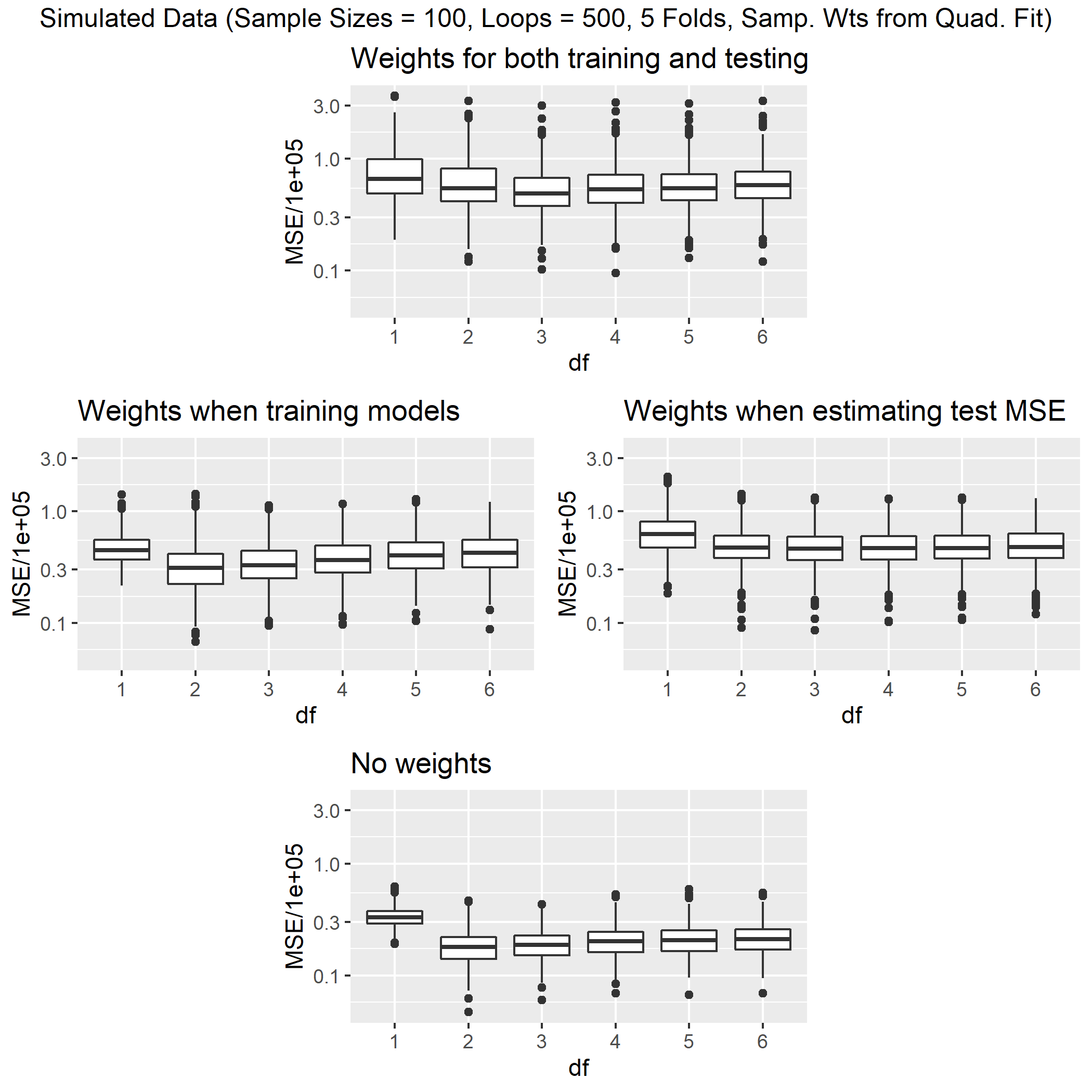# Plots for our surveyCVStat paper based on SDSS presentation

Writing cleaner code for only the simulations needed for our submission to Stat (the special issue for SDSS 2021 talks).

Based on the earlier sims (now on GitHub in data-raw/plot_generation.R and data-raw/early-results.html) – but instead of having similar functions repeatedly defined separately as in that file, use our functions such as cv.svy() directly from the package.

library(survey)
library(ggplot2)
library(splines)
library(magrittr)
library(surveyCV)
rerunSims <- FALSE  # when we *do* rerun sims, remember to reinstall package & restart R
knitr::opts_chunk$set(dpi=300, out.extra ='WIDTH="95%"') ## Generate the artificial population # Generate a cubic-ish artificial population with 1000 units set.seed(47) N <- 1000 nrStrata <- 10 nrClusters <- 100 clusSizes <- N/nrClusters x1 = stats::runif(1:(N/2), min = 26, max = 38) y1 = (x1-29)^3 - 13*(x1-29)^2 + 0*(x1-29) + 900 x2 = stats::runif(1:(N/2), min = 38, max = 50) y2 = (x2-36)^3 - 10*(x2-36)^2 + 2*(x2-36) + 600 z1 = jitter(y1, 15000) z2 = jitter(y2, 15000) ds1 <- data.frame(Response = z1, Predictor = x1) ds2 <- data.frame(Response = z2, Predictor = x2) ds12 <- rbind(ds1, ds2) b12 <- data.frame(ID = c(1:1000)) splinepop.df <- cbind(b12, ds12) splinepop.df <- splinepop.df %>% dplyr::arrange(Predictor) %>% # Create Cluster and Stratum variables so that # we can (separately) simulate both kinds of sampling dplyr::mutate(Stratum = dplyr::row_number(), Cluster = dplyr::row_number()) %>% dplyr::mutate(Stratum = cut(Stratum, nrStrata, 1:nrStrata), Cluster = cut(Cluster, nrClusters, 1:nrClusters)) %>% # Create fpc variables for each sampling type: # full pop has 1000 units; 100 units/stratum; 100 clusters (of 10 units each); # & if we added a combined Strat+Clus sim, we'd need different fpc's for that dplyr::mutate(fpcSRS = N, fpcStratum = N/nrStrata, fpcCluster = nrClusters) %>% dplyr::arrange(ID) # Create unequal sampling weights that are intentionally # NOT well matched to the true shape of the population, # so we can see how the use of weights impacts # model-training step vs test-error estimation step # in a case where naively ignoring weights will confidently pick wrong model lm_quad <- stats::lm(Response ~ Predictor + I(Predictor^2), data = splinepop.df) splinepop.df$samp_prob_quad <-
(1/(abs(lm_quad$residuals))) / sum(1/(abs(lm_quad$residuals)))
splinepop.df$samp_wt_quad <- 1/splinepop.df$samp_prob_quad

stopifnot(all.equal(sum(1/splinepop.df$samp_wt_quad), 1)) n <- 100 srs.df <- dplyr::sample_n(splinepop.df, n) stratcounts <- rep(n/nrStrata, nrStrata) names(stratcounts) <- 1:nrStrata s <- stratsample(splinepop.df$Stratum, stratcounts)
strat.df <- splinepop.df[s,]

c <- unique(splinepop.df[["Cluster"]])
clus.df <- splinepop.df[splinepop.df[["Cluster"]] %in% sample(c, n/clusSizes),]

a <- ggplot(splinepop.df, aes(x = Predictor, y = Response)) +
geom_point(shape = 1) +
labs(title = "Artificial Pop.", x = "Predictor", y = "Response") +
ylim(100,1650) + xlim(25, 51)
b <- ggplot(srs.df, aes(x = Predictor, y = Response)) +
geom_point(shape = 8, color = "darkgreen") +
labs(title = "SRS", x = "Predictor", y = "Response") +
ylim(100,1650) + xlim(25, 51)
c <- ggplot(strat.df, aes(x = Predictor, y = Response)) +
geom_point(shape = 3, color = "darkred") +
labs(title = "Stratified Sample", x = "Predictor", y = "Response") +
ylim(100,1650) + xlim(25, 51)
d <- ggplot(clus.df, aes(x = Predictor, y = Response)) +
geom_point(shape = 4, color = "steelblue") +
labs(title = "Cluster Sample", x = "Predictor", y = "Response") +
ylim(100,1650) + xlim(25, 51)
gridExtra::grid.arrange(a, b, c, d, ncol = 4,
top = "Simulated Data and Examples of How It Was Sampled")## Sims: Use of surveyCV folds with SRS, clustered, or stratified samples

# TODO: redo with different n's?
n <- 100
loops <- 500
# Use SRS samples and make SRS folds
srssrsds <- data.frame(df = c(), MSE = c())
# Use SRS samples and evaluate on full pop
srspopds <- data.frame(df = c(), MSE = c())

# Use Cluster samples and make Cluster folds
clusclusds <- data.frame(df = c(), MSE = c())
# Use Cluster samples and make SRS folds
clussrsds <- data.frame(df = c(), MSE = c())
# Use Cluster samples and evaluate on full pop
cluspopds <- data.frame(df = c(), MSE = c())

# Use Strat samples and make Strat folds
stratstratds <- data.frame(df = c(), MSE = c())
# Use Strat samples and make SRS folds
stratsrsds <- data.frame(df = c(), MSE = c())
# Use Strat samples and evaluate on full pop
stratpopds <- data.frame(df = c(), MSE = c())

modelsToFit <- c("Response~splines::ns(Predictor, df=1)",
"Response~splines::ns(Predictor, df=2)",
"Response~splines::ns(Predictor, df=3)",
"Response~splines::ns(Predictor, df=4)",
"Response~splines::ns(Predictor, df=5)",
"Response~splines::ns(Predictor, df=6)")

# Making as many simple random samples as we specify for 'loops'
for (i in 1:loops) {
# Take a SRS
sim.srs <- dplyr::sample_n(splinepop.df, n)

# Using our SRS function on SRS samples
srs.CV.out <- cv.svy(sim.srs, modelsToFit,
nfolds = 5, fpcID = "fpcSRS") %>% as.vector()
srssrsds2 <- data.frame(df = 1:6, MSE = srs.CV.out)

# Eval SRS samples on full pop
sub.srs <- dplyr::sample_n(sim.srs, n*.8)
srs.des <- svydesign(ids = ~1, fpc = ~fpcSRS, data = sub.srs)
srs.pop.out <- sapply(1:6,
function(ii) {
yhat <- predict(svyglm(modelsToFit[ii], srs.des),
newdata = splinepop.df)
return(mean((yhat - splinepop.df$Response)^2)) }) srspopds2 <- data.frame(df = 1:6, MSE = srs.pop.out) srspopds <- rbind(srspopds, srspopds2) } # Making as many cluster samples as we specify for 'loops' for (i in 1:loops) { # Take a Cluster sample c <- unique(splinepop.df[["Cluster"]]) sim.clus <- splinepop.df[splinepop.df[["Cluster"]] %in% sample(c, n/clusSizes),] # Using our Cluster function on Cluster samples clus.CV.out <- cv.svy(sim.clus, modelsToFit, clusterID = "Cluster", nfolds = 5, fpcID = "fpcCluster") %>% as.vector() clusclusds2 <- data.frame(df = 1:6, MSE = clus.CV.out) clusclusds <- rbind(clusclusds, clusclusds2) # Using our SRS function on Cluster samples srs.CV.out <- cv.svy(sim.clus, modelsToFit, nfolds = 5, fpcID = "fpcSRS") %>% as.vector() clussrsds2 <- data.frame(df = 1:6, MSE = srs.CV.out) clussrsds <- rbind(clussrsds, clussrsds2) # Eval 80% Cluster samples on full pop sub.c <- unique(sim.clus[["Cluster"]]) sub.clus <- sim.clus[sim.clus[["Cluster"]] %in% sample(sub.c, (n/clusSizes)*.8),] clus.des <- svydesign(ids = ~Cluster, fpc = ~fpcCluster, data = sub.clus) clus.pop.out <- sapply(1:6, function(ii) { yhat <- predict(svyglm(modelsToFit[ii], clus.des), newdata = splinepop.df) return(mean((yhat - splinepop.df$Response)^2))
})
cluspopds2 <- data.frame(df = 1:6, MSE = clus.pop.out)
cluspopds <- rbind(cluspopds, cluspopds2)
}

# Making as many stratified samples as we specify for 'loops'
for (i in 1:loops) {
# Take a Strat sample
stratcounts <- rep(n/nrStrata, nrStrata)
names(stratcounts) <- 1:nrStrata
s <- stratsample(splinepop.df$Stratum, stratcounts) sim.strat <- splinepop.df[s,] # Using our Strat function on Strat samples strat.CV.out <- cv.svy(sim.strat, modelsToFit, strataID = "Stratum", nfolds = 5, fpcID = "fpcStratum") %>% as.vector() stratstratds2 <- data.frame(df = 1:6, MSE = strat.CV.out) stratstratds <- rbind(stratstratds, stratstratds2) # Using our SRS function on Strat samples srs.CV.out <- cv.svy(sim.strat, modelsToFit, nfolds = 5, fpcID = "fpcSRS") %>% as.vector() stratsrsds2 <- data.frame(df = 1:6, MSE = srs.CV.out) stratsrsds <- rbind(stratsrsds, stratsrsds2) # Eval 80% Strat samples on full pop sub.stratcounts <- rep((n/nrStrata)*.8, nrStrata) names(sub.stratcounts) <- 1:nrStrata sub.s <- survey::stratsample(sim.strat$Stratum, sub.stratcounts)
sub.strat <- sim.strat[sub.s,]

strat.des <- svydesign(ids = ~1, strata = ~Stratum, fpc = ~fpcStratum, data = sub.strat)
strat.pop.out <- sapply(1:6,
function(ii) {
yhat <- predict(svyglm(modelsToFit[ii], strat.des),
newdata = splinepop.df)
return(mean((yhat - splinepop.df$Response)^2)) }) stratpopds2 <- data.frame(df = 1:6, MSE = strat.pop.out) stratpopds <- rbind(stratpopds, stratpopds2) } # Making the degrees of freedom variable a factor variable srssrsds$df <- as.factor(srssrsds$df) srspopds$df <- as.factor(srspopds$df) clusclusds$df <- as.factor(clusclusds$df) clussrsds$df <- as.factor(clussrsds$df) cluspopds$df <- as.factor(cluspopds$df) stratstratds$df <- as.factor(stratstratds$df) stratsrsds$df <- as.factor(stratsrsds$df) stratpopds$df <- as.factor(stratpopds$df) usethis::use_data(srssrsds, srspopds, clusclusds, clussrsds, cluspopds, stratstratds, stratsrsds, stratpopds, internal = FALSE, overwrite = TRUE) # TODO: for now setting internal=FALSE # so that we don't overwrite the sysdata.Rda for intro.Rmd; # but eventually when we're ready to replace that old vignette, # we can return to internal=TRUE here # if that's indeed better for R packages # ... # OK, now we've written those dataframes, # AND the 4 below, all into R/sysdata.Rda together if(!rerunSims) { srssrsds <- surveyCV:::srssrsds clusclusds <- surveyCV:::clusclusds clussrsds <- surveyCV:::clussrsds stratstratds <- surveyCV:::stratstratds stratsrsds <- surveyCV:::stratsrsds srspopds <- surveyCV:::srspopds cluspopds <- surveyCV:::cluspopds stratpopds <- surveyCV:::stratpopds } # Find the y-ranges of MSEs yminMSE <- min(min(srssrsds$MSE),
min(clusclusds$MSE), min(clussrsds$MSE),
min(stratstratds$MSE), min(stratsrsds$MSE))
ymaxMSE <- max(max(srssrsds$MSE), max(clusclusds$MSE), max(clussrsds$MSE), max(stratstratds$MSE), max(stratsrsds$MSE)) srspopds <- dplyr::group_by(srspopds, df) %>% dplyr::summarise(MSE = mean(MSE)) %>% dplyr::mutate(df = as.numeric(df)) cluspopds <- dplyr::group_by(cluspopds, df) %>% dplyr::summarise(MSE = mean(MSE)) %>% dplyr::mutate(df = as.numeric(df)) stratpopds <- dplyr::group_by(stratpopds, df) %>% dplyr::summarise(MSE = mean(MSE)) %>% dplyr::mutate(df = as.numeric(df)) # Making ggplot objects for the MSEs and AICs plot.srssrs <- ggplot(data = srssrsds, mapping = aes(x = df, y = MSE/1e4)) + geom_boxplot() + geom_line(data = srspopds, mapping = aes(x = df, y = MSE/1e4)) + ggtitle("CV: SRS folds,\nSRS sample") + scale_y_log10(limits = c(yminMSE, ymaxMSE)/1e4) plot.blank <- rectGrob(width = 0, height = 0) # empty spot here -- no corresponding sim plot.clusclus <- ggplot(data = clusclusds, mapping = aes(x = df, y = MSE/1e4)) + geom_boxplot() + geom_line(data = cluspopds) + ggtitle("CV: Cluster folds,\nCluster sample") + scale_y_log10(limits = c(yminMSE, ymaxMSE)/1e4) plot.clussrs <- ggplot(data = clussrsds, mapping = aes(x = df, y = MSE/1e4)) + geom_boxplot() + geom_line(data = cluspopds) + ggtitle("CV: SRS folds,\nCluster sample") + scale_y_log10(limits = c(yminMSE, ymaxMSE)/1e4) plot.stratstrat <- ggplot(data = stratstratds, mapping = aes(x = df, y = MSE/1e4)) + geom_boxplot() + geom_line(data = stratpopds) + ggtitle("CV: Strat folds,\nStrat sample") + scale_y_log10(limits = c(yminMSE, ymaxMSE)/1e4) plot.stratsrs <- ggplot(data = stratsrsds, mapping = aes(x = df, y = MSE/1e4)) + geom_boxplot() + geom_line(data = stratpopds) + ggtitle("CV: SRS folds,\nStrat sample") + scale_y_log10(limits = c(yminMSE, ymaxMSE)/1e4) gridExtra::grid.arrange(plot.srssrs, plot.clussrs, plot.stratsrs, plot.blank, plot.clusclus, plot.stratstrat, ncol = 3, top = paste0("Simulated Data (Sample Sizes = ", n, ", Clusters = ", n/clusSizes, " or Strata = ", nrStrata, ", Loops = ", loops, ", Folds = 5)"))## Sims: Use of sampling weights (in training model-fits vs in test loss-estimates) ggplot(splinepop.df, aes(x = Predictor, y = Response)) + geom_point(aes(size = samp_prob_quad), alpha = 0.1) + stat_smooth(method = "lm", formula = y ~ x + I(x^2), se = FALSE) + labs(title = "Artificial Population with Sampling Probabilities", subtitle = "Sampling prob. is higher for points nearer the solid curve", x = "Predictor", y = "Response", size = "Sampling Probability")# TODO: redo with different n's? n <- 100 loops <- 500 weights <- "samp_wt_quad" # In all the following cases, # we are only checking the effect of using sampling **weights** # during model-fitting on training sets, and testing on test sets; # we did not use cluster or stratified sampling, # so all CV folds will (sensibly) be SRS (regardless of samp weights) # Use weighted models, and calculate MSEs using weighted design AllW <- data.frame(df = c(), MSE = c()) # Use SRS models, and calculate MSEs using SRS design NoW <- data.frame(df = c(), MSE = c()) # Use weighted models, and calculate MSEs using SRS design ModW <- data.frame(df = c(), MSE = c()) # Use SRS models, and calculate MSEs using weighted design MSEW <- data.frame(df = c(), MSE = c()) for (i in 1:loops) { # Take a sample of size n, using the sampling probabilities instead of SRS # (using 1/samp_wt as the samp_prob per unit, # or n/samp_wt as overall samp_prob to get a sample of size n) in.sample <- sampling::UPtille(n / splinepop.df[[weights]]) splinesamp.df <- splinepop.df[in.sample > 0, ] modelsToFit <- c("Response~ns(Predictor, df=1)", "Response~ns(Predictor, df=2)", "Response~ns(Predictor, df=3)", "Response~ns(Predictor, df=4)", "Response~ns(Predictor, df=5)", "Response~ns(Predictor, df=6)") AllWdat <- cv.svy(splinesamp.df, modelsToFit, nfolds = 5, weightsID = weights) %>% as.vector() NoWdat <- cv.svy(splinesamp.df, modelsToFit, nfolds = 5) %>% as.vector() ModWdat <- cv.svy(splinesamp.df, modelsToFit, nfolds = 5, weightsID = weights, useSvyForLoss = FALSE) %>% as.vector() MSEWdat <- cv.svy(splinesamp.df, modelsToFit, nfolds = 5, weightsID = weights, useSvyForFits = FALSE) %>% as.vector() # compiling one data frame AllW2 <- data.frame(df = 1:6, MSE = AllWdat, sample = rep(i, 6)) AllW <- rbind(AllW, AllW2) NoW2 <- data.frame(df = 1:6, MSE = NoWdat, sample = rep(i, 6)) NoW <- rbind(NoW, NoW2) ModW2 <- data.frame(df = 1:6, MSE = ModWdat, sample = rep(i, 6)) ModW <- rbind(ModW, ModW2) MSEW2 <- data.frame(df = 1:6, MSE = MSEWdat, sample = rep(i, 6)) MSEW <- rbind(MSEW, MSEW2) } # Making the degrees of freedom variable a factor variable AllW$df <- as.factor(AllW$df) NoW$df <- as.factor(NoW$df) MSEW$df <- as.factor(MSEW$df) ModW$df <- as.factor(ModW$df) usethis::use_data(AllW, NoW, MSEW, ModW, internal = FALSE, overwrite = TRUE) # TODO: as above, for now setting internal=FALSE # so that we don't overwrite the sysdata.Rda for intro.Rmd; # but eventually when we're ready to replace that old vignette, # we can return to internal=TRUE here # if that's indeed better for R packages # ... # OK, now we've written those dataframes, # AND the 8 above, all into R/sysdata.Rda together if(!rerunSims) { AllW <- surveyCV:::AllW NoW <- surveyCV:::NoW MSEW <- surveyCV:::MSEW ModW <- surveyCV:::ModW } # Find the y-range of MSEs ymin <- min(min(AllW$MSE), min(NoW$MSE), min(MSEW$MSE), min(ModW$MSE)) ymax <- max(max(AllW$MSE), max(NoW$MSE), max(MSEW$MSE), max(ModW\$MSE))
# Making a ggplot object for the MSEs collected when using
# SRS folds, SRS models, and SRS error calculations
pAllW <- ggplot(data = AllW, mapping = aes(x = df, y = MSE/1e5)) +
ggtitle("Weights for both training and testing") +
scale_y_log10(limits = c(ymin, ymax)/1e5) + geom_boxplot()
# Making a ggplot object for the MSEs collected when using
# SRS folds, Clus models, and SRS error calculations
pNoW <- ggplot(data = NoW, mapping = aes(x = df, y = MSE/1e5)) +
ggtitle("No weights") +
scale_y_log10(limits = c(ymin, ymax)/1e5) + geom_boxplot()
# Making a ggplot object for the MSEs collected when using
# Clus folds, Clus models, and Clus error calculations
pModW <- ggplot(data = ModW, mapping = aes(x = df, y = MSE/1e5)) +
ggtitle("Weights when training models") +
scale_y_log10(limits = c(ymin, ymax)/1e5) + geom_boxplot()
# Making a ggplot object for the MSEs collected when using
# Clus folds, SRS models, and Clus error calculations
pMSEW <- ggplot(data = MSEW, mapping = aes(x = df, y = MSE/1e5)) +
ggtitle("Weights when estimating test MSE") +
scale_y_log10(limits = c(ymin, ymax)/1e5) + geom_boxplot()

# Making a grid display of the four plot objects above
lay <- matrix(c(NA, 1, 1, NA,
NA, 1, 1, NA,
2, 2, 3, 3,
2, 2, 3, 3,
NA, 4, 4, NA,
NA, 4, 4, NA), byrow = TRUE, ncol = 4)
gridExtra::grid.arrange(pAllW, pModW, pMSEW, pNoW, ncol = 2,
top = paste0("Simulated Data (Sample Sizes = ", n,
", Loops = ", loops, ", 5 Folds, Samp. Wts from Quad. Fit)"),
layout_matrix = lay)